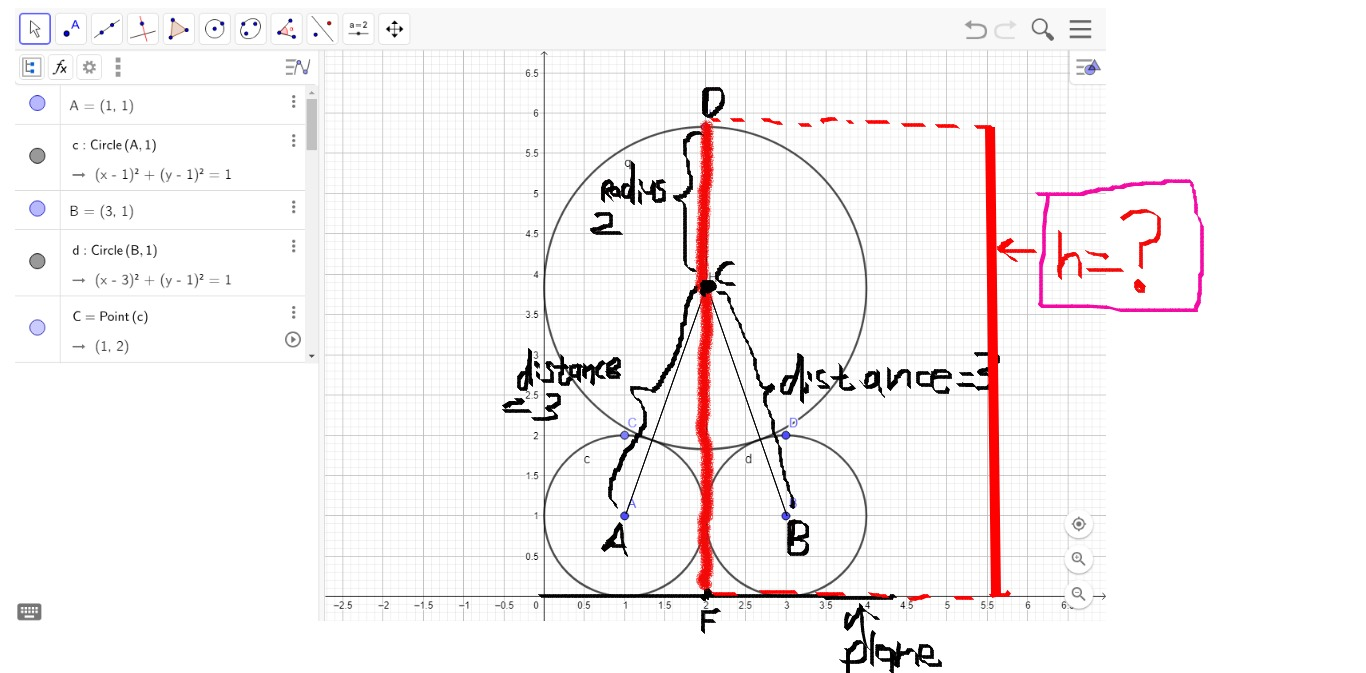# The question is below?

## # mutually tangent spheres of radius 1 rest on a horizontal plane. A sphere of radius 2 rests on them. What is the distance from the plane to the top of larger sphere?

Jun 28, 2018

The distance is $2 \setminus \sqrt{2} + 3$ or $\setminus \approx 5.828427125$

#### Explanation:

Let the radiuses of the mutually tangent spheres be $A \left(1 , 1\right)$ and $B \left(3 , 1\right)$
respectively

Let $C \left(2 , y\right)$ be the radius of the sphere that lies on top of them$\overline{A C} = 3$ and $\overline{B C} = 3$
Recall the distance formula:
$d = \sqrt{{\left({x}_{1} - {x}_{2}\right)}^{2} + {\left({y}_{1} - {y}_{2}\right)}^{2}}$

Thus,
${1}^{2} + {\left(y - 1\right)}^{2} = {3}^{2}$
$1 + {\left(y - 1\right)}^{2} = 9$
${\left(y - 1\right)}^{2} = 8$
$y = 1 \setminus \pm 2 \sqrt{2}$

Since $y$ must be greater than 1 because it is on top of the spheres, reject the negative solution
$y = 2 \sqrt{2} + 1$

Since the radius of the sphere is 2, $h = y + 2$
height $h = y + 2 = 2 \sqrt{2} + 1 + 2$

$\setminus \therefore$ The distance is $2 \setminus \sqrt{2} + 3$